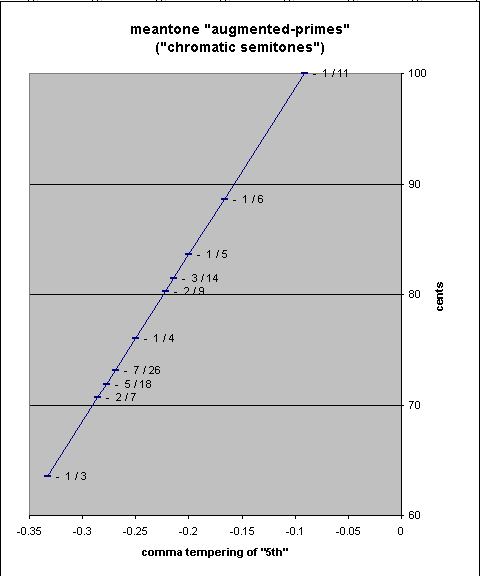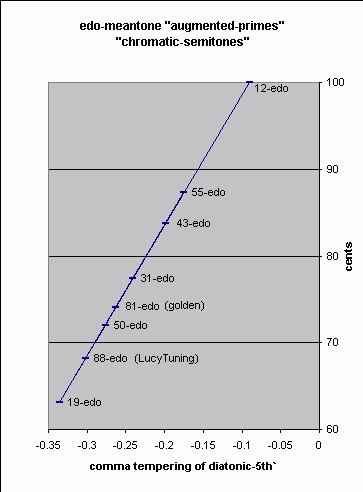# chromatic semitone / augmented-prime / #1

[Joe Monzo]

A diatonic interval which is the difference between the tone (i.e., whole-tone) and the diatonic semitone. In several different theories of the medieval, Renaissance, Baroque, and Classical periods, a distinction was made between chromatic and diatonic semitones, as there were two different sizes of semitone in many tuning systems. (Two good examples are pythagorean and meantone.)

The chromatic semitone is the interval between two notes of a scale which both have the same nominal (letter-names in the case of the traditional theory explained here), but which have different accidentals (including the natural), for example, A - A#. The implication is that only one of the two is a bonafide diatonic scale degree. This interval is called the "augmented prime" and may be found abbreviated as aug1, #1, or +1 .

The chromatic semitone arose out of ancient Greek tetrachord theory, by the fact that the Perfect Immutable System includes both the Lesser Perfect System (with its tetrachord synemmenon) and the Greater Perfect System (with its tetrachord diezeugmenon). When medieval theorists applied the Roman alphabet to musical notation, designating mese as "A", the paramese at the bottom of tetrachord diezeugmenon became "B" a whole-tone above A, but the trite synemmenon at the bottom of tetrachord synemmenon became a different B only a semitone above A -- we now call it Bb. Our modern accidentals grew out of these two different forms of B, and the chromatic semitone is the difference between them.

```              - E  nete diezeugmenon
|
tetrachord  |  D  paranete diezeugmenon        D  nete synemmenon    -
diezeugmenon |                                                         | tetrachord
|  C  trite diezeugmenon           C  paranete synemmenon | synemmenon
- B  paramese                                            |
tone of     <                                Bb  trite synemmenon    |
disjunction     A  mese                         A  mese               -
```

The interval-sizes may be shown schematically thus ("t" = tone, "s" = diatonic semitone, "(t-s)" = chromatic semitone):

```       t
______________
|              |
s     (t-s)    s
A     Bb       B     C
|______________|
t
```

In the pythagorean [3-limit] system, the chromatic semitone is also called the apotome.

Note that in positive systems (typical example: pythagorean tuning), the chromatic semitone is larger than the diatonic, while in negative systems (typical example: any meantone tuning) it is exactly the opposite and the diatonic is the larger.

Typical meantone chromatic semitones range in size from about 63 to 100 cents. Below is a table and pitch-height graph of the size of the chromatic semitone for several historical meantones:

```meantone           adjustment of 81/64
"flavor"    --- [pythagorean 'major 3rd'] ---     ----- 2,3,5-monzo -----      ~ cents

1/11-comma   ((3/2)7) / (22) / ((81/80)(7*1/11)) =  [-93/11  49/11,   7/11 >   =  99.99918541
1/6-comma    ((3/2)7) / (22) / ((81/80)(7*1/6))  =  [-19/3    7/3 ,   7/6  >   =  88.59433486
1/5-comma    ((3/2)7) / (22) / ((81/80)(7*1/5))  =  [-27/5    7/5 ,   7/5  >   =  83.57620062
3/14-comma   ((3/2)7) / (22) / ((81/80)(7*3/14)) =  [ -5/1    1/1 ,   3/2  >   =  81.42557166
2/9-comma    ((3/2)7) / (22) / ((81/80)(7*2/9))  =  [-43/9    7/9 ,  14/9  >   =  80.2307778
1/4-comma    ((3/2)7) / (22) / ((81/80)(7*1/4))  =  [ -4/1    0   ,   7/4  >   =  76.04899926
7/26-comma   ((3/2)7) / (22) / ((81/80)(7*7/26)) =  [-45/13  -7/13,  49/26 >   =  73.15392182
5/18-comma   ((3/2)7) / (22) / ((81/80)(7*5/18)) =  [-29/9   -7/9 ,  35/18 >   =  71.86722073
2/7-comma    ((3/2)7) / (22) / ((81/80)(7*2/7))  =  [ -3     -1   ,   2    >   =  70.67242686  [the ratio 25:24]
1/3-comma    ((3/2)7) / (22) / ((81/80)(7*1/3))  =  [ -5/3   -7/3 ,   7/3  >   =  63.50366367
```Note that the JI ratio [ -3 -1, 2 > = 25:24 is rendered exactly in 2/7-comma meantone, and that another JI ratio which falls within this range, and which also vanishes in meantone (because it is a syntonic-comma larger than 25:24), is [ -3 -1, 2 > + [ -4 4, -1 > = [-7 3, 1 > = 135:128 = ~ 92.17871646 cents.

Below is a table and pitch-height graph of the size of the chromatic semitone for several edo-meantones:

```  edo     ratio of
meantone  generator         ~cents

12-edo    2( 7/12)   100
55-edo    2(32/55)    87.27272727
43-edo    2(25/43)    83.72093023
31-edo    2(18/31)    77.41935484
81-edo    2(47/81)    74.07407407  (~ golden meantone)
50-edo    2(29/50)    72
88-edo    2(51/88)    68.18181818  (~ LucyTuning)
19-edo    2(11/19)    63.15789474
```Marchetto of Padua specified three types of semitone and called the third, naturally enough, the enharmonic; however, his names were matched with the intervals differently than in traditional theory.

. . . . . . . . .

The tonalsoft.com website is almost entirely the work of one person: me, Joe Monzo. Please reward me for my knowledge and effort by choosing your preferred level of financial support. Thank you.

 support level donor: \$5 USD friend: \$25 USD patron: \$50 USD savior: \$100 USD angel of tuning: \$500 USD microtonal god: \$1000 USD